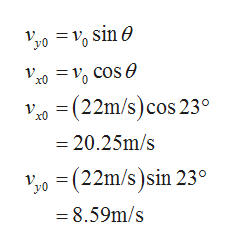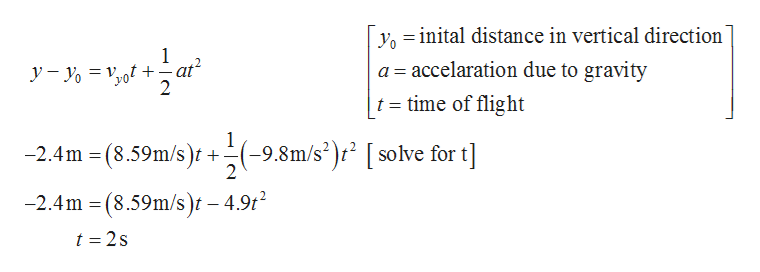# A golf ball is struck with an initial velocity of 22 m/s at an angle of 23 degrees above the horizontal. On its downward trajectory, the ball hits an elevated green 2.4 meters above the tee elevation. Determine the flight time in seconds.Determine the horizontal distance traveled (meters) by the golf ball under the conditions described above.Determine the magnitude of the golf ball velocity (m/s) when it first strikes the green under the conditions described above.

Question
57 views

A golf ball is struck with an initial velocity of 22 m/s at an angle of 23 degrees above the horizontal. On its downward trajectory, the ball hits an elevated green 2.4 meters above the tee elevation. Determine the flight time in seconds.

Determine the horizontal distance traveled (meters) by the golf ball under the conditions described above.

Determine the magnitude of the golf ball velocity (m/s) when it first strikes the green under the conditions described above.

check_circle

Step 1

The vertical and horizontal component of velocity is given by,help_outlineImage Transcriptionclose= ", sin 0 =10 cos e = (22m/s)cos 23° 20.25m/s (22m/s)sin 23 8.59m/s fullscreen
Step 2

The relation of the vertical distance and time of flight ishelp_outlineImage Transcriptioncloseyoinital distance in vertical direction 1 accelaration due to gravity y-yo t +-at 2 a = t time of flight +(9.8m/s2)r [solve for t -2.4m (8.59m/s)t -2.4m (8.59m/s)t - 4.9t2 t 2s fullscreen
Step 3

The horizontal distance traveled by...

### Want to see the full answer?

See Solution

#### Want to see this answer and more?

Solutions are written by subject experts who are available 24/7. Questions are typically answered within 1 hour.*

See Solution
*Response times may vary by subject and question.
Tagged in
SciencePhysics

### Newtons Laws of Motion